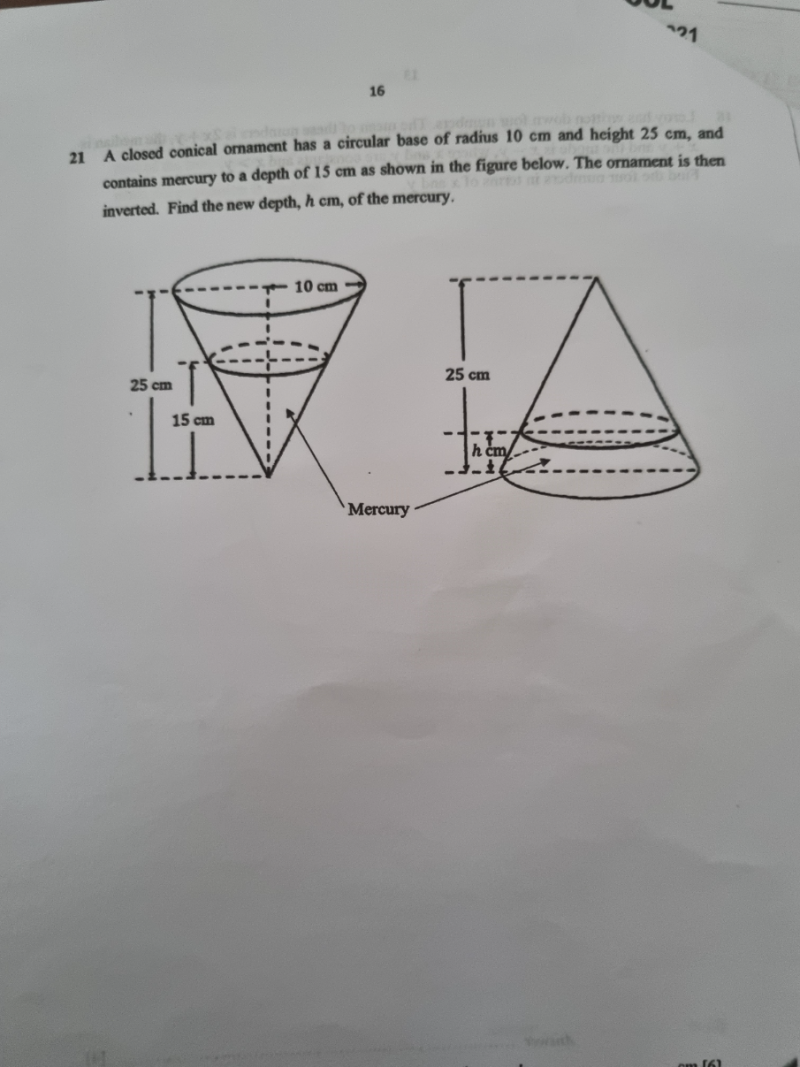# QuestionThank you

Let the small radius in diagram 1 be r1

r1 /10 = 15/25

r1= (3/5) x 10 = 6

Volume of truncated cone = (1/3) * π * h * (r² + r * R + R²)

Volume with “no mercury” truncated cone in the diagram 1 = (1/3) * π * (10) * (6² + 6 * 10 + 10²) = (1960/3 ) π

Let the new small radius in diagram 2 be r

(25-h) / 25 = r/10
25 – h = 25(r/10)
h = 25 – (5/2)r

If h = 25- (5/2)r =>  height of  “no mercury cone”  in diagram 2 = (5/2)r

Volume of the no mercury cone in diagram 2 = ⅓ 𝜋r2h = ⅓ 𝜋r2 (5/2)r

⅓ 𝜋r2 (5/2)r   =  (1960/3 ) π
(5/2) r3  = 1960
r3  =  (1960 x 2) / 5 = 784
r = ∛784

Hence h = 25 – (5/2)∛784  = 1.95cm ( correct to 2 dec places)
0 Replies 1 Like ✔Accepted Answer